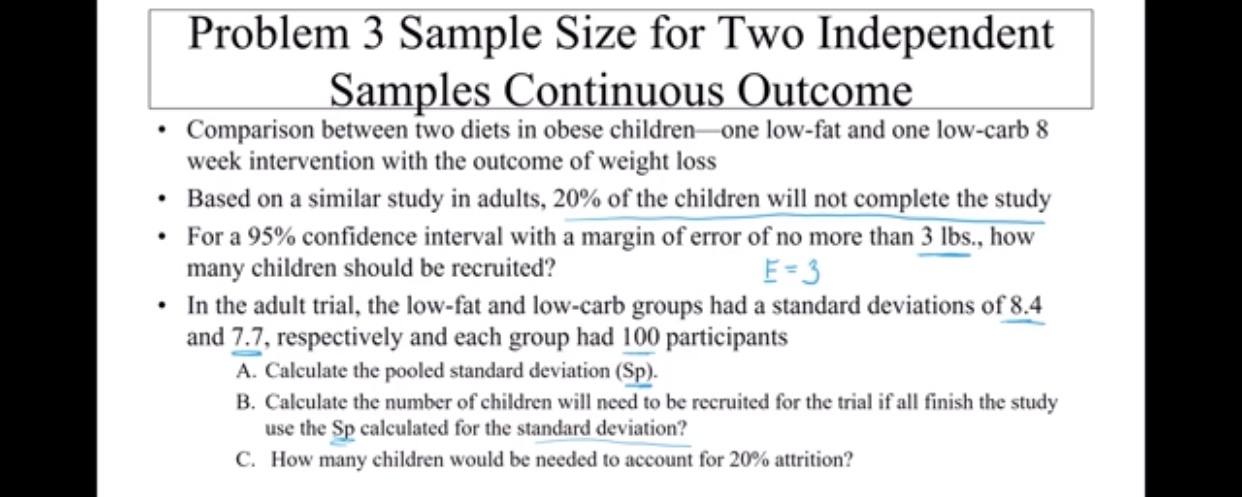# Problem 3 Sample Size for Two Independent Samples Continuous Outcome Comparison between two diets in obese children one low-fat and one low-carb 8 week intervention with the outcome of weight loss Based on a similar study in adults, 20 % of the children will not complete the study For a 95% confidence interval with a margin of error of no more than 3 lbs., how many children should be recruited? In the adult trial, the low-fat and low-carb groups had a standard deviations of 8.4 and 7.7, respectively and each group had 100 participants A. Calculate the pooled standard deviation (Sp) B. Calculate the number of children will need to be recruited for the trial if all finish the study use the Sp calculated for the standard deviation? C. How many children would be needed to account for 20% attrition? E-3

Question

Problem 3help_outlineImage TranscriptioncloseProblem 3 Sample Size for Two Independent Samples Continuous Outcome Comparison between two diets in obese children one low-fat and one low-carb 8 week intervention with the outcome of weight loss Based on a similar study in adults, 20 % of the children will not complete the study For a 95% confidence interval with a margin of error of no more than 3 lbs., how many children should be recruited? In the adult trial, the low-fat and low-carb groups had a standard deviations of 8.4 and 7.7, respectively and each group had 100 participants A. Calculate the pooled standard deviation (Sp) B. Calculate the number of children will need to be recruited for the trial if all finish the study use the Sp calculated for the standard deviation? C. How many children would be needed to account for 20% attrition? E-3 fullscreen

1 Rating

### Want to see this answer and more?

Experts are waiting 24/7 to provide step-by-step solutions in as fast as 30 minutes!*

*Response times vary by subject and question complexity. Median response time is 34 minutes and may be longer for new subjects.
Tagged in
MathStatistics

### Other